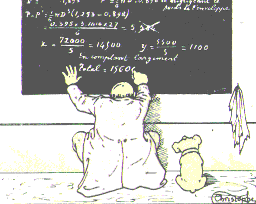Let $X={(X_t)}_{t\geq0}$ be an irreducible Markov process with generator $G$ and unique invariant law $\mu$. What is the difference between irreversible and out of equilibrium? If you do not know what is a generator, they you may replace it by a transition kernel without loss of interpretation.

Trajectories. A Markov process is a way to transform an initial law into a law of trajectory, by mean of a generator. The law of the trajectory ${(X_t)}_{t\geq0}$ depends on the law of $X_0$ as well as on the generator $G$. The equilibrium or invariant law $\mu$ depends only on $G$.

Reversibility. We say that $X$ is reversible when $X_0\sim\mu$ implies that for all $t\geq0$ the trajectory $(X_s)_{0\leq s\leq t}$  and the reversed trajectory ${(X_{t-s})}_{0\leq s\leq t}$ have same law. This means that if we start the process from its equilibrium, the notion of orientation of time is not visible. Up to regularity considerations, this is equivalent to say that the generator is a symmetric operator in $L^2(\mu)$. When $X$ is a finite Markov chain, this corresponds to the detailed balance condition $\mu(x)G(x,y)=\mu(y)G(y,x)$ for all $x,y$ in the state space. A famous theorem by Kolmogorov states that this is equivalent to say that the weight of all cycles with respect to the transition kernel or generator does not depend on the orientation of the cycle, and the remarkable fact about this criterion is that it does not involve $\mu$.

Out of equilibrium. We say that the process $X$ is out of equilibrium when it is not started from the equilibrium $\mu$ meaning that $X_0\not\sim\mu$. Up to regularity assumptions, for all initial law, the process converges in law to its equilibrium in long time, in other words $\lim_{t\to\infty}X_t=\mu$ in law. On the contrary, if $X_0\sim\mu$, then the process satisfies $X_t\sim\mu$ for all $t\geq0$ since $\mu$ is invariant, and we say in this case that the process is at equilibrium.

Conclusion. Being out of equilibrium or not has nothing to do with being reversible or not. We may start a reversible process or not, out of equilibrium, or not. If we start a reversible process out of equilibrium, then typically it will converge exponentially fast to its equilibrium $\mu$ in $L^2(\mu)$, and the exponential speed of this convergence is given by the spectral gap of $G$.

This site uses Akismet to reduce spam. Learn how your comment data is processed.

Syntax · Style · .